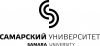# Questions to the Instructor

3 сообщения / 0 новоеSamara University
Не в сети
Questions to the Instructor

Here you can ask questions concerning the theme of the course and the content of the videos.Selenium
Не в сети

Hello! This course is very interesting and I would like to ask the quetions about some themes.

In chapter 1 we had to study out why 5-fold rotation axis are not possible in crystalography. So I read that it is not possible because expression 2cos(2pi/n) must be an integer. n is equal to 1,2,3,4 and 6. But why exist this expression? Where does it come from?

The second question. Why phisical properties have symmetry and what is it? Will we study this theme next chapters?

I will be grateful if you help meSamara University
Не в сети

Hello! We're glad to hear it!

1. You can find the asnwer here.

2. Depending on their nature, forces and fields have their symmetry. Let's take the gravity as an example. The gravity can be considered as a force vector directed towards the core of the Earth, and one can say then it has the symmetry of a vector, which belong to the point group of a cone Cnv (where n is infinite). The gravity exerts its symmetry into each object on the Earth, which means, for instance, that one cannot grow a perfect crystal of, say, D2h point group. Moreover, there is no symmetry higher than Cnv among living creatures.